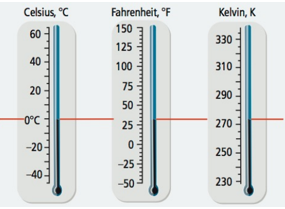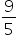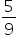Mathematics
Easy

Question

# The temperature in degrees Celsius is 32 less than the Fahrenheit temperature, multiplied by five ninths. The temperature in degree Kelvin is the number of degrees Celsius plus 273.Identify the conversion formula for finding the number of degrees Kelvin, given the temperature in Fahren he it.

## K =(F - 32) + 273 K =(F - 32)K =(F + 32) - 273K =(F - 32) + 273Hint:

## The correct answer is: K =(F - 32) + 273

### Related Questions to study#### With Turito Foundation.#### Get an Expert Advice From Turito.